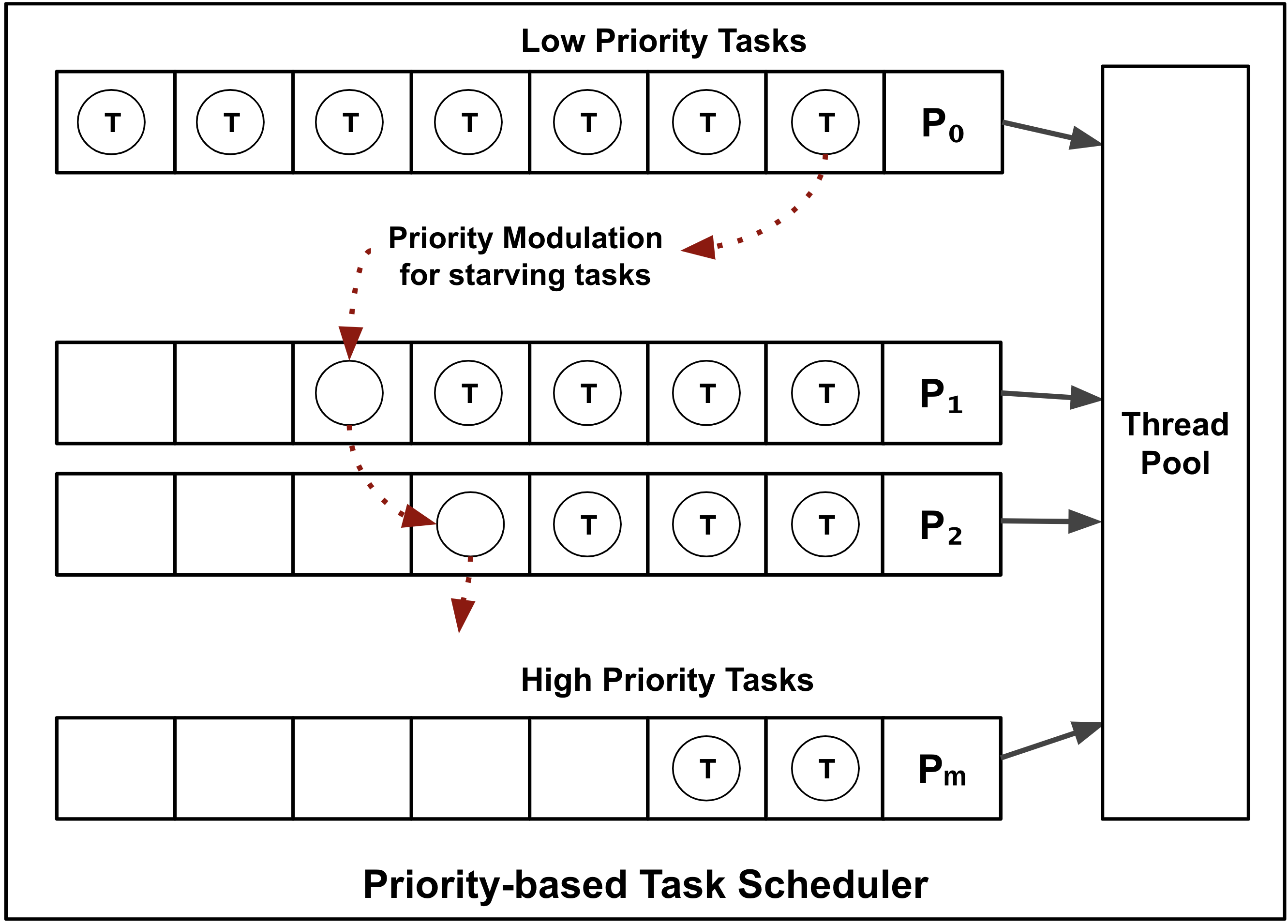# psched

`psched` is a lightweight library that provides a priority-based task scheduler for modern C++.

• The `psched` scheduler manages an array of concurrent queues, each queue assigned a priority-level
• A task, when scheduled, is enqueued onto one of queues based on the task's priority
• The priority of starving tasks is modulated based on the age of the task## Getting Started

Consider the task set below. There are three periodic tasks: `a`, `b` and `c`.

Task Period (ms) Burst Time (ms) Priority
a 250 130 0 (Lowest)
b 500 390 1
c 1000 560 2 (Highest)

Here, burst time refers to the amount of time required by the task for executing on CPU.

### Create a `PriorityScheduler`

First, let's create a scheduler:

``````#include <iostream>
#include <psched/priority_scheduler.h>
using namespace psched;

int main() {
aging_policy<
increment_priority_by<1>
>>
scheduler;
``````

### Create a `Task`

Create the first task, `Task a` like below. The task "performs work" for 130ms. The post-completion callback, called when the task has completed, can be used to study the temporal behavior of the task, e.g., waiting time, burst time, and turnaround time.

``````

std::cout << "Waiting time = " << stats.waiting_time() << "ms; ";
std::cout << "Burst time = " << stats.burst_time() << "ms; ";
std::cout << "Turnaround time = " << stats.turnaround_time() << "ms\n";
}
);
``````

Now, we can write a simple periodic timer that schedules this task at `priority<0>`:

``````  auto timer_a = std::thread([&scheduler, &a]() {
while (true) {

scheduler.schedule<priority<0>>(a);

// Sleep for 250ms and repeat
}
});
``````

We can repeat the above code for tasks `b` and `c`:

``````  Task b(
std::cout << "Waiting time = " << stats.waiting_time() << "ms; ";
std::cout << "Burst time = " << stats.burst_time() << "ms; ";
std::cout << "Turnaround time = " << stats.turnaround_time() << "ms\n";
});

auto timer_b = std::thread([&scheduler, &b]() {
while (true) {
scheduler.schedule<priority<1>>(b);
}
});

std::cout << "Waiting time = " << stats.waiting_time() << "ms; ";
std::cout << "Burst time = " << stats.burst_time() << "ms; ";
std::cout << "Turnaround time = " << stats.turnaround_time() << "ms\n";
});

auto timer_c = std::thread([&scheduler, &c]() {
while (true) {
scheduler.schedule<priority<2>>(c);
}
});

timer_a.join();
timer_b.join();
timer_c.join();
}
``````

Running this sample may yield the following output:

``````./multiple_periodic_tasks
[Task a] Waiting time = 0ms; Burst time = 133ms; Turnaround time = 133ms
[Task c] Waiting time = 0ms; Burst time = 563ms; Turnaround time = 563ms
[Task b] Waiting time = 0ms; Burst time = 395ms; Turnaround time = 395ms
[Task a] Waiting time = 60ms; Burst time = 134ms; Turnaround time = 194ms
[Task a] Waiting time = 0ms; Burst time = 131ms; Turnaround time = 131ms
[Task b] Waiting time = 0ms; Burst time = 390ms; Turnaround time = 390ms
[Task a] Waiting time = 3ms; Burst time = 135ms; Turnaround time = 139ms
[Task a] Waiting time = 0ms; Burst time = 132ms; Turnaround time = 132ms
[Task b] Waiting time = 8ms; Burst time = 393ms; Turnaround time = 402ms
[Task c] Waiting time = 0ms; Burst time = 561ms; Turnaround time = 561ms
[Task a] Waiting time = 6ms; Burst time = 133ms; Turnaround time = 139ms
[Task b] Waiting time = [Task a] Waiting time = 0ms; Burst time = 393ms; Turnaround time = 393ms
0ms; Burst time = 133ms; Turnaround time = 133ms
[Task a] Waiting time = 11ms; Burst time = 134ms; Turnaround time = 145ms
[Task a] Waiting time = 0ms; Burst time = 134ms; Turnaround time = 134ms
[Task b] Waiting time = 11ms; Burst time = 394ms; Turnaround time = 405ms
[Task c] Waiting time = 0ms; Burst time = 560ms; Turnaround time = 560ms
[Task a] Waiting time = 7ms; Burst time = 132ms; Turnaround time = 139ms
[Task b] Waiting time = 0ms; Burst time = 390ms; Turnaround time = 390ms
[Task a] Waiting time = 0ms; Burst time = 130ms; Turnaround time = 130ms
[Task a] Waiting time = 17ms; Burst time = 130ms; Turnaround time = 148ms
[Task a] Waiting time = 0ms; Burst time = 131ms; Turnaround time = 131ms
[Task b] Waiting time = 10ms; Burst time = 390ms; Turnaround time = 401ms
[Task c] Waiting time = 0ms; Burst time = 560ms; Turnaround time = 560ms
``````

## Building Samples

``````git clone https://github.com/p-ranav/psched
cd psched
mkdir build && cd build
cmake -DPSCHED_SAMPLES=ON ..
make
``````

``````python3 utils/amalgamate/amalgamate.py -c single_include.json -s .
``````

## Contributing

Contributions are welcome, have a look at the CONTRIBUTING.md document for more information.

The project is available under the MIT license.

Get A Weekly Email With Trending Projects For These Topics
No Spam. Unsubscribe easily at any time.
C Plus Plus (370,121
Cpp17 (1,809
Queue (1,710
Lightweight (1,366
Concurrency (1,291